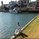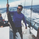# Volume Accumulation Percentage Indicator [LazyBear]

13496 views
13496
Volume Accumulation Percentage Indicator (VAPI) is a variation of the classic volume-accumulation indicators. It uses volume the same way as OBV except that it assigns volume weights based on intraday volatility .

In a positive trend, if the stock price is close to a (local) new high, the VAPI should be at its maximum as well (and vice versa for a negative trend). If the market is looking for a direction, if the price is in the bottom side of his current trading range and if, at the same moment the VAPI osc slopes up, there is accumulation on the stock and that the prices should start to climb. The SELL signal is given when the VAPI decreases (distribution). Divergences are usually very effective too.

Tuning the "length" parameter may be needed for your instrument (default is 10), do let me know if you find a different value to be optimal for majority of instruments.

List of my public indicators: http://bit.ly/1LQaPK8
List of my app-store indicators: http://blog.tradingview.com/?p=970
```//
// @author LazyBear
// List of my public indicators: http://bit.ly/1LQaPK8
// List of my app-store indicators: http://blog.tradingview.com/?p=970
//
study("Volume Accumulation Percentage Indicator [LazyBear]", shorttitle="VAPI_LB", overlay=false)
length=input(10, title="Time Periods")
x=(2*close-high-low)/(high-low)
tva=sum(volume*x,length)
tv=sum(volume,length)
va=100*tva/tv
zl=plot(0, color=gray, title="ZeroLine")
vac=gray
val=plot(va, color=vac, style=histogram, title="VAPI Histogram")
us=va<0?0:(va==0?va  :va), ls=va>0?0:(va==0?va:va)
ux=plot(us, color=gray, style=circles, title="DummyU"), lx=plot(ls, color=gray, style=circles, title="DummyL")
fill(ux,val,color=red, transp=50, title="NegativeFill"), fill(lx,val,color=lime, transp=50, title="PositiveFill")
plot(va, color=gray, linewidth=2, title="VAPI Osc")```as usual..this is awesome.. I have been looking for a package of 3 indies.. looks like this is one, 2 is SMIIO, 3 still looking for..